2022: SklogWiki celebrates 15 years on-line

# Berendsen barostat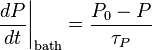$\left. \frac{dP}{dt} \right\vert_{\mathrm {bath} } = \frac{P_0 - P}{\tau_P}$
where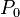$P_0$ is the reference pressure, i.e. the pressure of the external pressure "bath", and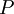$P$ is the instantaneous pressure.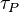$\tau_P$ is a time constant. Within this scheme the coordinates and the box sides are rescaled every step. Assuming the system is isotropic and within a cubic box the scaling factor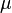$\mu$ is given by (Eq. 20):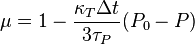$\mu = 1 - \frac{\kappa_T \Delta t}{3\tau_P} (P_0 -P)$
where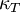$\kappa_T$ is the isothermal compressibility. The value of$\kappa_T$ only has to be reasonable; for example, both DL POLY and GROMACS use the value of the compressibility of water (at 1 atm and 300K, leading to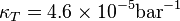$\kappa_T = 4.6 \times 10^{-5} \mathrm{bar}^{-1}$).